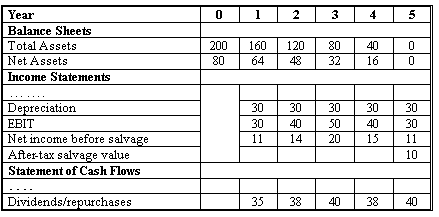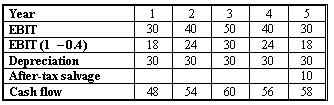### Seeing is believing!

Before you order, simply sign up for a free user account and in seconds you'll be experiencing the best in CFA exam preparation.

### Subject 7. Other Income Measures and Valuation Models

Economic and Accounting Income

• Economic income is the investment's after-tax cash flow plus the change in the market value.
• Accounting income is revenues minus expenses.

Economic income = Cash flow + Change in market value
= Cash flow - economic depreciation

Economic income and accounting income are usually different for a couple of reasons:

• Depreciation.

• Accounting depreciation, based on the original cost of the investment, is the decrease in the market value of the investment.
• Economic depreciation is the decrease in the market value of the investment.

Since the accounting depreciation is not based on the market value of the investment, the accounting depreciation schedule does not follow the declines in the market value of the investment.

• Interest expense.
In computing economic income, interest expenses are ignored. However, accounting net income is net of the after-tax interest expenses on the company's debt obligations.

Economic Profit

The concept of economic profit (EP) is that a firm only adds value for its shareholders if it makes a profit in excess of what could have been earned if its capital were invested elsewhere. The basic formula is

EP = NOPAT - opportunity cost of capital

• NOPAT denotes net operating profit after taxes. It is an accounting concept. Firms report it in their financial statements.
• The opportunity cost of capital is usually WACC x capital. It reflects the expected return on capital obtainable from other, similarly risky venture. This approach is from the perspective of all suppliers of capital.
• A positive economic profit indicates that a firm has added value for shareholders.

Residual Income

Residual income for a given period is the earnings for that period excess of the investors' required rate of return on beginning-of-period investment (common stockholders' equity). That is, it equals accounting earnings minus opportunity costs of invested capital.

Residual income = Net income - Equity charge,
or
RIt = NIt - re x Bt - 1

where
NIt is the net income during period t.
re is the required rate of return on equity.
Bt - 1 is the beginning-of-period book value of equity.

• The approach adds the present value of residual income to the original debt and equity investments in the company.
• It takes the perspective of equity investors so residual income is discounted at the cost of equity.
• Residual income attempts to match profits to the time period in which they are earned but not necessarily realized as cash.

Example

Suppose shareholders' initial investment is \$100 million. The required rate of return on the stock is 10%. The company earns \$12 million in the course of a year. How much value has the company added for shareholders?

A return of 0.1 x \$100 million = \$10 million just meets the amount investors could have earned in an equivalent-risk investment. Only the residual excess amount of \$12 million - \$10 million = \$2 represents value added, or an economic gain, to shareholders. So, \$2 million is the company's residual income for the period.

Claims Valuation

This approach values an asset by valuing the claims against the asset. For example, an asset financed with debt and equity has a value equal to the value of the debt plus the value of the equity.

A Comprehensive Example

The financial statements for a company are shown below (in thousands):The company is going out of business in five years, distributing its income to bondholders and equity holders.

• Cost of equity: 16%.
• Before-tax cost of debt: 8%.
• Tax rate: 40%.
• Debt/Equity ratio: 50/50.

1. Calculate the economic income during year 1.

First we calculate its WACC: 0.16 x 50% + 0.08 x 50% x (1 - 0.4) = 10.4%.Economic income (year 1) = Cash flow - Economic depreciation = CF1 - (V0 - V1).
CF1 = 48.
V0 = 48/1.1041 + 54/1.1042 + 60/1.1043 + 56/1.1044 + 58/1.1045 = 205.4374
V1= 54/1.1041 + 60/1.1042 + 56/1.1043 + 58/1.1044 = 178.8029
Economic income (year 1) = 48 - (205.4374 - 178.8029) = 21.3655

2. Calculate the EP during year 1.

EP = NOPAT - opportunity cost of capital = NOPAT - WACC x total capital = EBIT x (1 - tax rate) - WACC x total capital = 30 x (1 - 0.4) - 0.104 x 200 = -2.8.

3. Calculate the residual income during year 1.

RIt = NIt - re x Bt - 1 = 11 - 0.16 x 80 = -1.8.

4. Calculate the value of equity at time 0.

It is the PV of cash distributions to equity.
PV = 35/1.161 + 38/1.162 + 40/1.163 + 38/1.164 + 40/1.165 = 124.0705.

#### Practice Question 1

The WACC for a capital project is 10%. The after-tax operating cash flows are \$10,000 each year for the next 2 years. The project is expected to have a 2-year-life and 0 salvage value. The economic income of the project during year 1 is:

A. \$9,091
B. \$1,735
C. not enough information.